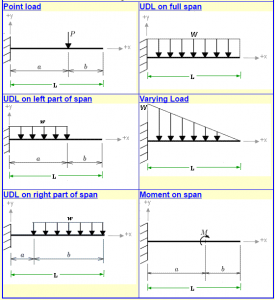# Calculator for deflection and slope of cantilever

Slope and deflection of structures are required to be calculated for many reasons. There are many methods for finding slope and deflection of beams. Calculation for deflection and slope of cantilever is made easy by using the calculator given below which can solve the problem for six different loading cases of cantilever i.e. point load, uniform load varying load and pure moment acting on the cantilever.

Principle of superposition can be used for finding the deflection due to more than one loading case.

This calculator absolutely Free and available at the following link;

https://civilengineer.webinfolist.com/str/sdcalc2.htmREAD  Calculator for Deflection and slope of Simply Supported Beam